#Function Repository Resource:

# PolynomialHomogenize

Homogenize a polynomial with respect to a given set of variables

Contributed by: Daniel Lichtblau
 ResourceFunction["PolynomialHomogenize"][poly,vars,t] homogenizes the expanded polynomial poly in total degree of vars using the new variable t. ResourceFunction["PolynomialHomogenize"][{poly1,poly2,…},vars,t] homogenizes each of polyi.

## Details and Options

Each term in ResourceFunction["PolynomialHomogenize"][poly,vars,t] will have the same total degree. Terms in poly of lower degree are augmented by an appropriate power of t.

## Examples

### Basic Examples

Homogenize the equation for a hyperbola:

 In:=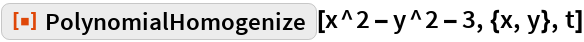Out=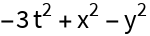Create a homogenized polynomial of total degree 4:

 In:=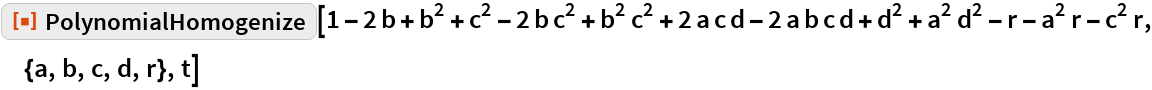Out=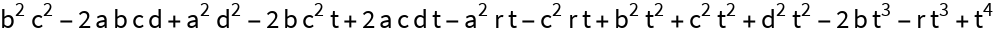### Scope

Any variables appearing in a polynomial that are not specified in vars are treated as parameters (that is, part of the coefficients):

 In:=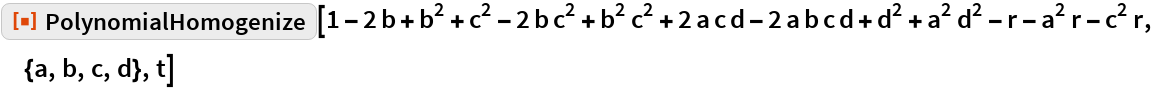Out=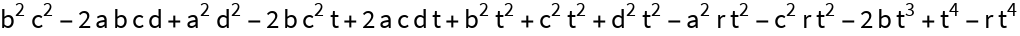### Properties and Relations

A polynomial can be dehomogenized by setting the homogenizing variable to unity:

 In:=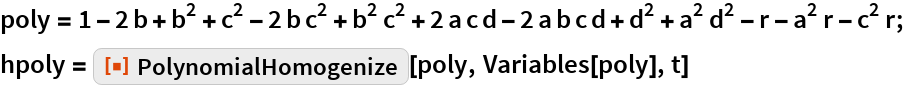Out=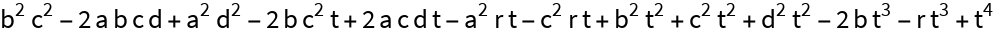In:=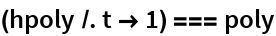Out=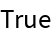### Possible Issues

PolynomialHomogenize requires the input polynomial to be fully expanded:

 In:=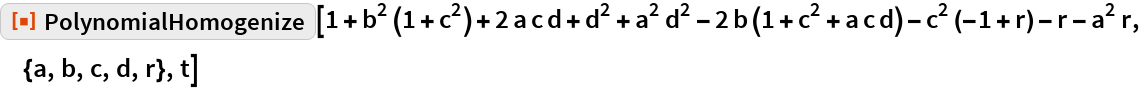Out=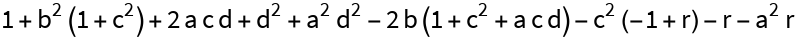## Requirements

Wolfram Language 11.3 (March 2018) or above